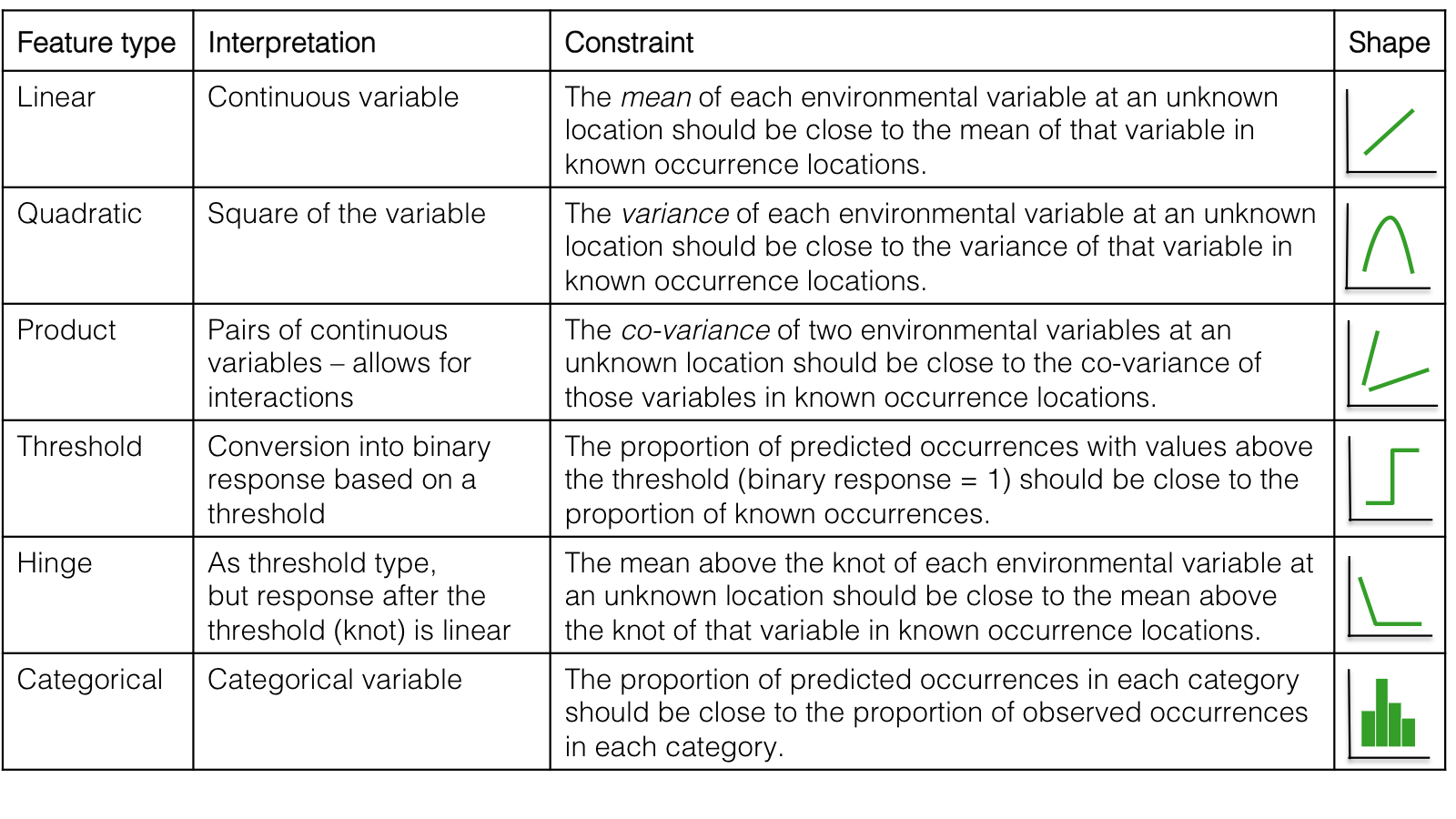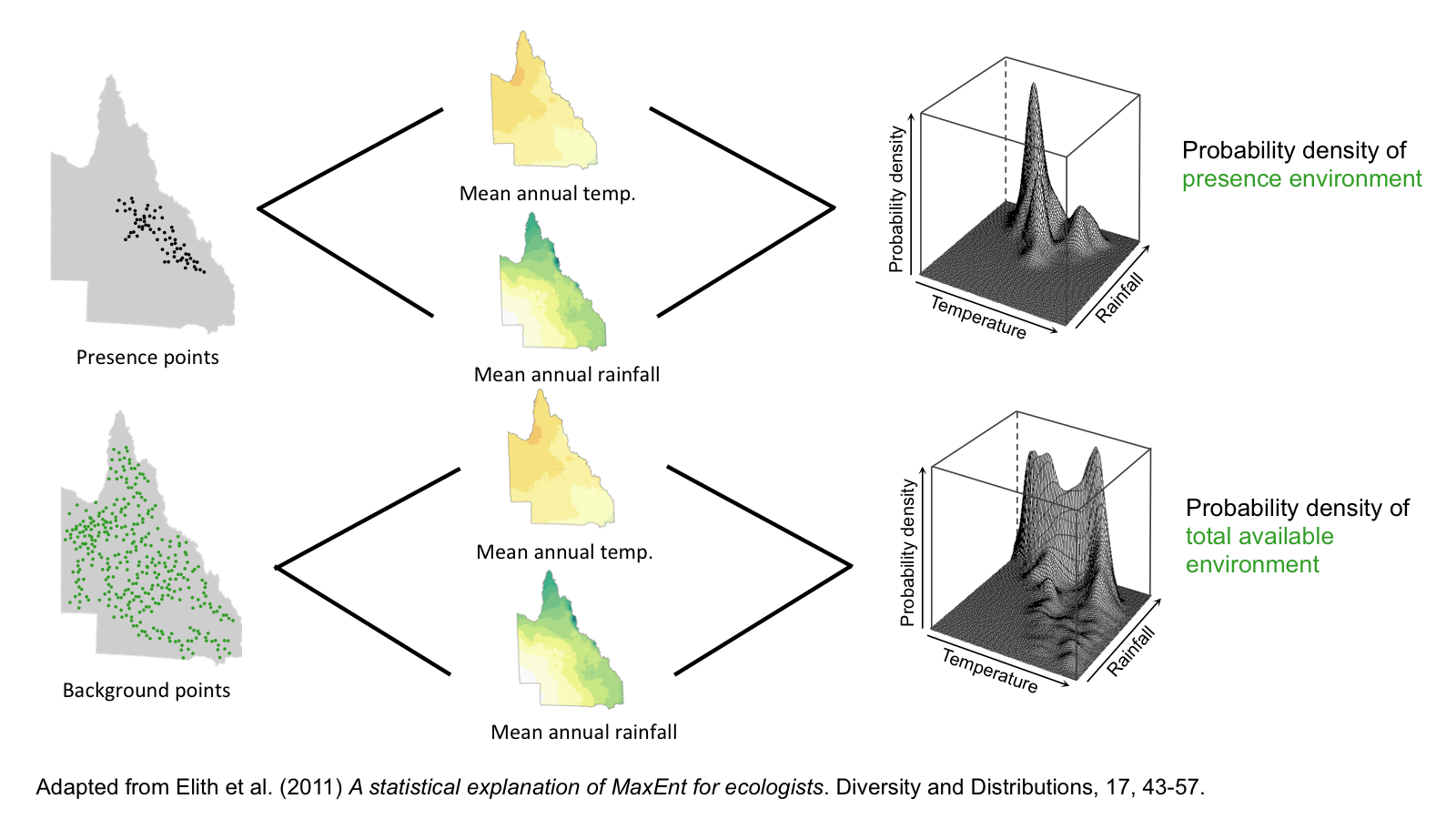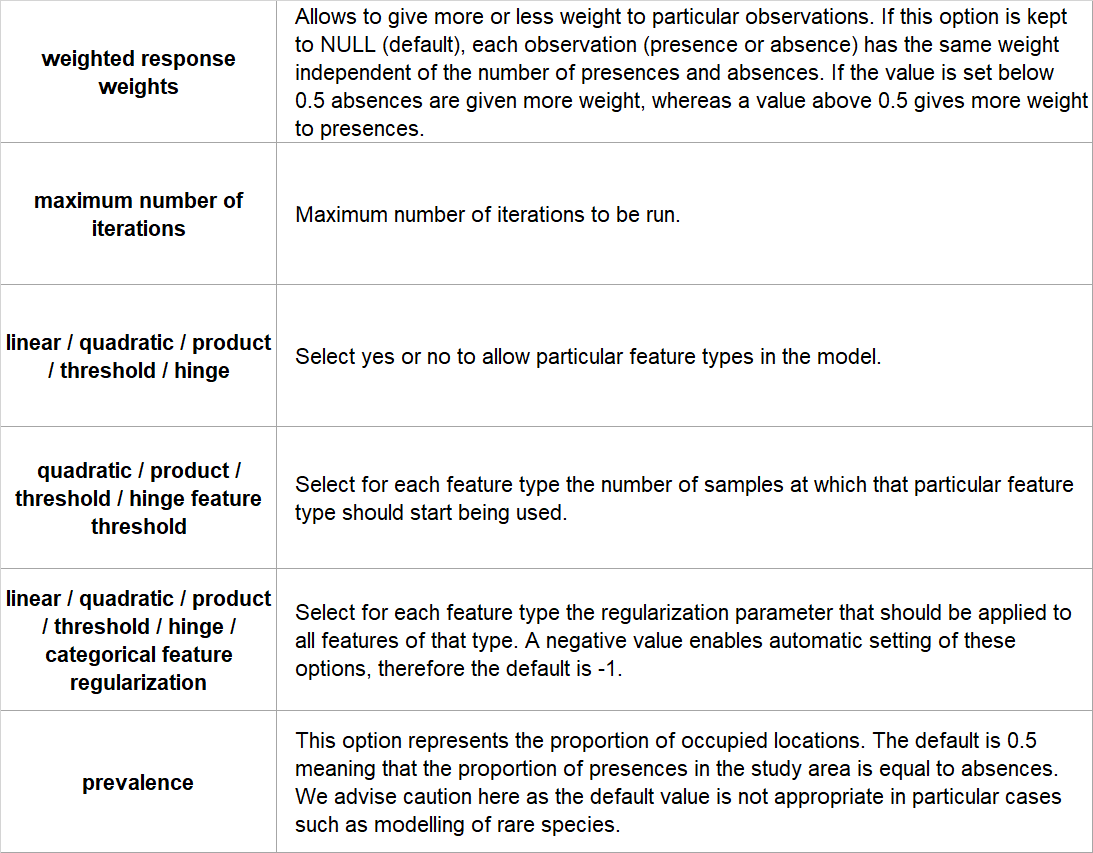### Introduction

Maxent, which stands for maximum entropy modelling, predicts species occurrences by finding the distribution that is most spread out, or closest to uniform, while taking into account the limits of the environmental variables of known locations.

Maxent only uses presence data and the algorithm compares the locations of where a species has been found to all the environments that are available in the study region. It defines these available environments by sampling a large number of points throughout the study area, which are referred to as background points. Because background points can include locations where the species is known to occur, background points are not the same as pseudo-absence points. Background points define the available environment.

The Maxent algorithm developed for species distribution modelling is a machine learning method, and thus iteratively builds multiple models. It has two main components:

1. Entropy: the model is calibrated to find the distribution that is most spread out, or closest to uniform throughout the study region.

2. Constraints: the rules that constrain the predicted distribution. These rules are based on the values of the environmental variables (called features) of the locations where the species has been observed.

Maxent considers six types of features, and each of these types allows a different possible shape of the response curves, and has different implications for the constraints. As a default Maxent uses all feature types, but you can choose to build simpler models by only using a few of these.To calculate the potential distribution of a species, Maxent first calculates two probability densities. For all presence points, the probability density describes the relative likelihood of all environmental variables in the model over the range of those points. For example, in the figure below the values for temperature and rainfall under the peak in the graph on the right were the most common values across all values of the presence environment. Similarly, a probability density is calculated across the entire study region based on the background points. Thus, the probability density of the background points characterizes the available environment within the study region, whereas the probability density of the presence points characterizes the environment of where a species has been found. Maxent then calculates the ratio between these two probability densities, which gives the relative environmental suitability for presence of a species for each point in the study area.Maxent chooses the distribution that maximizes the similarity between the environmental characteristics of the total environment and those of the locations where the species is known to be present. This is known as the raw output of Maxent. For easier interpretation of the results, and to provide an estimate of the probability that a species is present in a given location, Maxent performs a logistic transformation of the raw output. The logistic output takes into account the prevalence of a species, which refers to the proportion of occupied locations. Maxent uses a default prevalence value of 0.5, which implies that the species is present in half of all the possible locations. We advise to be cautious with this default value as the exact prevalence cannot be derived from presence-only data, and a value of 0.5 is for example not appropriate for rare species.

An important aspect of Maxent is regularization, which reduces overfitting of the model. Regularization is done in two ways:

1. Relaxing the constraints: instead of fitting the model using the exact constraints (means, variances etc) of the environmental variables, it takes into account confidence intervals around the constraints. This prevents the model from being fitted to closely around the input data.

2. Penalizing complexity: the model excludes feature types that do not add a significant improvement to the model.

• Requires only presence data
• Can use both continuous and categorical predictor variables
• Includes interactions between predictor variables
• Includes a regularization protocol to protect against overfitting
• Generally shows good predictive performance

### Limitations

• Difficult to compare output with other algorithms, as Maxent output gives environmental suitability rather than predicted probability of occurrence
• Maxent's logistic output relies on an assumption, not an estimation, of prevalence

### Assumptions

Maxent by default assumes that prevalence is 0.5, which is not always appropriate.

No.

### Configuration options

BCCVL uses the Maxent software (http://www.cs.princeton.edu/~schapire/maxent/), implemented in biomod2. The user can set the following configuration options:### References

• Elith J, Phillips SJ, Hastie T, Dudík M, Chee YE, Yates CJ (2011) A statistical explanation of MaxEnt for ecologists. Diversity and Distributions, 17(1): 43-57.
• Guillera‐Arroita G, Lahoz‐Monfort JJ, Elith J (2014) Maxent is not a presence–absence method: a comment on Thibaud et al. Methods in Ecology and Evolution, 5(11), 1192-1197.
• Merow C, Smith MJ, Silander JA (2013) A practical guide to MaxEnt for modeling species’ distributions: what it does, and why inputs and settings matter. Ecography, 36(10), 1058-1069.
• Phillips SJ, Anderson RP, Schapire RE (2006) Maximum entropy modeling of species geographic distributions. Ecological modelling, 190(3): 231-259.
• Phillips SJ, Dudík M (2008) Modeling of species distributions with Maxent: new extensions and a comprehensive evaluation. Ecography, 31(2): 161-175.
• Thibaud E, Petitpierre B, Broennimann O, Davison AC, Guisan A (2014) Measuring the relative effect of factors affecting species distribution model predictions. Methods in Ecology and Evolution, 5(9), 947-955.
• Yackulic CB, Chandler R, Zipkin EF, Royle JA, Nichols JD, Campbell Grant EH, Veran S (2013) Presence‐only modelling using Maxent: when can we trust the inferences? Methods in Ecology and Evolution, 4(3), 236-243.